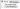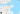Click on the banner to learn about and purchase my database training on Azure

# SQL Server - How to calculate the distance between two locations using latitude and longitude (without API)

Views: 5.252

Hey guys!

In this post, I will show you how to calculate the distance between two locations using latitude and longitude (without API). In March of 2017, I demonstrated how to do this using the Google Maps API in the post. SQL Server - How to calculate the distance between two points using the Google API (zip code, address or latitute and longitude)but in today's post the distance will be calculated using only math and geometry

This calculation, instead of using an API, can be especially useful for calculating the distance between a large volume of geographic points (the Google API has limitations on the number of queries per day in the free plan).

It is important to note that the calculation without API considers a straight line between 2 points, while the Google API takes into account the routes and routes, according to the chosen mode of transport, which may have values ​​closer to the real, of according to necessity, and quite different from geometric calculation.

## Introduction

As you know, the measurements we currently use to identify the geographical location of a point on the globe are latitude and longitude, which can be expressed in two ways:

• Latitude: -20.3222, Longitude: -40.3381
• 20 ° 19 ′ 20 ″ South, 40 ° 20 ′ 17 ″ West

Our position on the earth is referenced to the equator line and the Greenwich meridian and is expressed in two values: latitude and longitude. So to know our position on Earth just know latitude and longitude.

Latitude is the distance to the equator measured along the Greenwich meridian. This distance is measured in degrees and may range from 0º to 90º to North (N) or South (S). Longitude is the distance to the Greenwich meridian measured along the equator. This distance is measured in degrees and may vary from 0º to 180º to the east (E) or to the west (W).

To identify the latitude and longitude of a location, you can use several shapes. One is using Google Maps, as shown below:## Calculating the distance between two latitudes and longitudes

To perform this calculation, I will use the haversine formula, an important equation used in navigation, providing distances between two points of a sphere from its latitudes and longitudes, having as its mathematical basis, the Cosine Law, considering in the model the curvature of the Earth, that is, the radius of the Earth. has a value of approximately 6.371 km or 3.959 miles.

For demonstration of this calculation, I will use the latitude and longitude I sent in the example above (Shopping Vitória / -20.3135958, -40.2893737) and compare with the coordinates of Shopping Vila Velha (-20.3480338, -40.2975204) using the function proposed in this article, which will return the distance between the two points in KM.Comparing with Google Maps, we can see that the calculated distance is very close:The code for this function is available here:

It is noteworthy that I added an adjustment percentage of 15% in value to bring the distance closer to the real.

That's it folks!
I hope you enjoyed this post.

A hug and see you next.